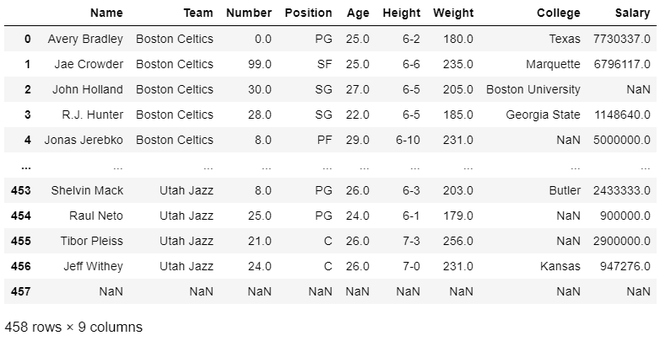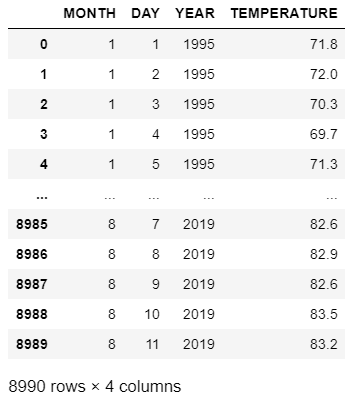Convert CSV to Pandas Dataframe

• Difficulty Level : Medium
• Last Updated : 02 Dec, 2020

In this article, we will discuss how to convert CSV to Pandas Dataframe, this operation can be performed using pandas.read_csv reads a comma-separated values (csv) file into DataFrame.

Example 1: In the below program we are going to convert nba.csv into a data frame and then display it.

Python

 # import pandas module import pandas as pd     # making dataframe df = pd.read_csv("nba.csv")    # output the dataframeprint(df)

Output:Example 2: Here is another example to convert a CSV dataset into pandas data frame.

Python

 # import pandas module import pandas as pd     # making dataframe df = pd.read_csv("nba.csv")    # output the dataframeprint(df)

Output:My Personal Notes arrow_drop_up